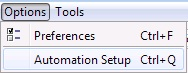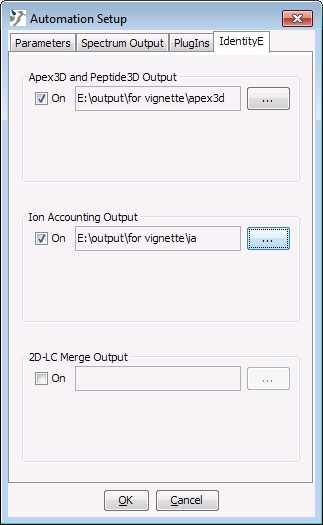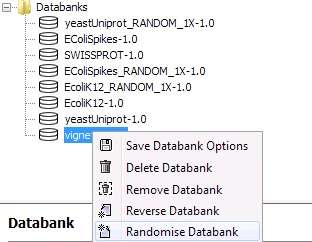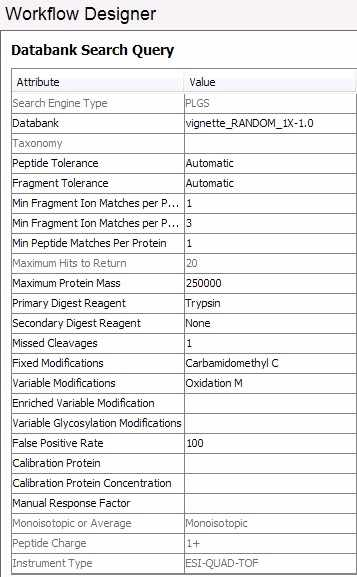# Foreword

synapter is free and open-source software. If you use it, please support the project by citing it in publications:

Nicholas James Bond, Pavel Vyacheslavovich Shliaha, Kathryn S. Lilley, and Laurent Gatto. Improving qualitative and quantitative performance for MS$$^E$$-based label free proteomics. J. Proteome Res., 2013, 12 (6), pp 2340–2353

# Questions and bugs

For bugs, typos, suggestions or other questions, please file an issue in our tracking system (https://github.com/lgatto/synapter/issues) providing as much information as possible, a reproducible example and the output of sessionInfo().

If you don’t have a GitHub account or wish to reach a broader audience for general questions about proteomics analysis using R, you may want to use the Bioconductor support site: https://support.bioconductor.org/.

# 1 Introduction

## 1.1 Background

The main functionality of synapter is to combine proteomics data acquired under different mass spectrometry settings or with different samples to (i) optimise the respective qualities of the two data sets or (ii) increase the number of identifications, thereby decreasing missing values. Besides synapter offers other functionality inaccessible in the default pipeline, like peptide FDR estimation and filtering on peptide match type and peptide uniqueness.

The example that motivated the development of this package was to combine data obtained on a Synapt G2 instrument:

1. HDMS$$^E$$ data, acquired with additional peptide separation using an ion mobility cell, thus leading to better (both in number and in quality) identification and
2. standard MS$$^E$$ data (acquired without ion mobility separation), providing better data quantitation.

The former is data is called identification peptides and the latter quantitation peptides, irrespective of the acquisition mode (HDMS$$^E$$ or MS$$^E$$). This HDMS$$^E$$/MS$$^E$$ design is used in this document to illustrate the synapter package.

However, although HDMS$$^E$$ mode possesses superior identification and MS$$^E$$ mode superior quantitation capabilities and transferring identifications from HDMS$$^E$$ to MS$$^E$$ is a priori the most efficient setup, identifications can be transferred between any runs, independently of the acquisition mode. This allows to reduce the number of missing values, one of the primary limitation of label-free proteomics. Thus users will benefit from synapter’s functionality even if they run their instruments in a single mode (HDMS$$^E$$ or MS$$^E$$ only).

However, as will be shown in section Data analysis, transferring identifications from multiple runs to each other increases analysis time and peptide FDR within the analysis. synapter allows to minimise these effects to acceptable degree by choosing runs to transfer identifications from and merging them in the master HDMS$$^E$$ file.

This data processing methodology is described in section HDMS$$^E$$/MS$$^E$$ data analysis and the analysis pipeline is described in section Different pipelines.

To maximise the benefit of combining better identification and quantitation data, it is also possible to combine several, previously merged identification data files into one master set. This functionality is described in section Using master peptide files.

Finally, section Analysis of complex experiments illustrates a complete pipeline including synapter and MSnbase (Gatto and Lilley 2012) packages to perform protein label-free quantitation: how to combine multiple synapter results to represent the complete experimental design under study and further explore the data, normalise it and perform robust statistical data analysis inside the R environment.

The rationale underlying synapter’s functionality are described in (Shliaha et al. 2013) and (Bond et al. 2013). The first reference describes the benefits of ion mobility separation on identification and the effects on quantitation, that led to the development of synapter, which in described and demonstrated in (Bond et al. 2013).

synapter is written for R~(R Core Team 2012), an open source, cross platform, freely available statistical computing environment and programming language. Functionality available in the R environment can be extended though the usage of packages. Thousands of developers have contributed packages that are distributed via the Comprehensive R Archive Network (CRAN) or through specific initiatives like the Bioconductor project (Gentleman et al. 2004), focusing on the analysis and comprehension of high-throughput biological data.

synapter is such an R package dedicated to the analysis of label-free proteomics data. To obtain detailed information about any function in the package, it is possible to access it’s documentation by preceding it’s name with a question mark at the command line prompt. For example, to obtain information about the synapter package, one would type ?synapter.

## 1.2 Installation

synapter is available through the Bioconductor project. Details about the package and the installation procedure can be found on its page. Briefly, installation of the package and all its dependencies should be done using the dedicated Bioconductor infrastructure as shown below:

if (!require("BiocManager"))
install.packages("BiocManager")
BiocManager::install("synapter")

After installation, synapter will have to be explicitly loaded with

library("synapter")

so that all the package’s functionality is available to the user.

# 2 Data analysis using synapter

## 2.1 Preparing the input data

Preparation of the data for synapter requires the .raw data first to be processed with Waters’ ProteinLynx Global Serve (PLGS) software. The PLGS result is then exported as csv spreadsheet files in user specified folders. These csv files can then be used as input for synapter.

We also highly recommend users to acquaint themselves with the PLGS search algorithm for data independent acquisitions (Li et al. 2009).

First the user has to specify the output folders for files to be used in synapter analysis as demonstrated in the figures. After the folders are specified ignore the message that appears requiring restarting PLGS.At the first stage PLGS performs noise reduction and centroiding, based on user specified preferences called processing parameters. These preferences determine thresholds in intensity for discriminating between noise peaks and peptide and fragment ion peaks in high and low energy functions of an acquisition. The optimal value of these parameters is sample dependant and different between MS$$^E$$ and HDMS$$^E$$ modes. For synapter to function properly all acquisitions in the analysis have to be processed with the same thresholds, optimal for the mode identifications are transferred from (typically HDMS$$^E$$ mode). The user is expected to identify optimal parameters himself for every new sample type by repeatedly analysing a representative acquisition with different thresholds.

After the ions peaks have been determined and centroided, the ions representing charge states and isotopes of a peptide are collapsed into a single entity called EMRT (exact mass retention time pair). The EMRTs in low energy function represent unidentified peptides and are assigned peptides sequences during database search. The total list of EMRTs can be found in the pep3DAMRT.csv file and it is one of the synapter input files for the runs used for quantitation (typically MS$$^E$$ mode)

Prior to the database search, randomised entries are added to the database to allow PLGS to compute protein false positive rate. The randomised entries can either be added automatically or manually, using the Randomise Databank function in the Databank admin tool. To properly prepare the files for synapter, the user has to add randomised entries manually via Databank admin tool, since only then randomised entries identified in the database search will be displayed in the csv output. The following figures demonstrate how to create a randomised databank manually using one randomised entry per regular entry.The user is also expected to use a minimum of 1 fragment per peptide, 3 fragments per protein and 1 peptide per protein identification thresholds and 100% False Positive Rate4 This is erroneously termed false positive rate in the software and manuscript and should be considered a false discovery rate. for protein identification during database search for all of the acquisitions in the analysis as demonstrated in figure. This allows to maximise the number of identified peptides from the randomised part of the database, needed to estimate peptide identifications statistics. The total list of identified peptides is given in final_peptide.csv files. A single final_peptide.csv file has to be supplied to synapter for every run in the analysis (for both identification and quantitation runs).

More details and screenshots are available in a separate document available at https://lgatto.github.com/synapter/.

## 2.2 HDMS$$^E$$/MS$$^E$$ data analysis

The analysis of pairs of HDMS$$^E$$ and MS$$^E$$ data files is based on the following rationale – combine strengths or each approach by matching high quality HDMS$$^E$$ identifications to quantified MS$$^E$$ EMRTs applying the following algorithm:

1. Apply various peptide filters to HDMS$$^E$$ and MS$$^E$$ peptides to obtain two sets of reliably identified unique proteotypic peptides.
2. Use shared HDMS$$^E$$ and MS$$^E$$ peptides to model the deviations in retention time between the two mass spectrometer runs.
3. Optimise the parameters that will be used to optimally match all HDMS$$^E$$ peptides and quantified MS$$^E$$ EMRTs using a grid search.
4. Using the best parameters, match identified HDMS$$^E$$ peptides to quantified MS$$^E$$ EMRTs.

## 2.3 Different pipelines

Two different pipeline are available to the user:

### 2.3.1 Wrapper function

The synergise function is a high level wrapper that implements a suggested analysis to combine two files (see next paragraph for details). A set of parameters can be passed, although sensible defaults are provided. While the analysis is executed, a html report is created, including all result files in text spreadsheet (csv format) and binary R output. This level allows easy scripting for automated batch analysis. Using data from the synapterdata package, the following code chunk illustrates the synergise usage. An example report can be found online at https://lgatto.github.com/synapter/.

library("synapterdata")
hdmsefile <- getHDMSeFinalPeptide()
basename(hdmsefile)
##  "HDMSe_101111_25fmol_UPS1_in_Ecoli_04_IA_final_peptide.csv.gz"
msefile <- getMSeFinalPeptide()
basename(msefile)
##  "MSe_101111_25fmol_UPS1_in_Ecoli_03_IA_final_peptide.csv.gz"
msepep3dfile <- getMSePep3D()
basename(msepep3dfile)
##  "MSe_101111_25fmol_UPS1_in_Ecoli_03_Pep3DAMRT.csv.gz"
fas <- getFasta()
basename(fas)
##  "EcoliK12_enolase_UPSsimga_NB.fasta"
## the synergise input is a (named) list of filenames
input <- list(identpeptide = hdmsefile,
quantpeptide = msefile,
quantpep3d = msepep3dfile,
fasta = fas)
## a report and result files will be stored
## in the 'output' directory
output <- tempdir()
output
##  "/tmp/RtmplTY2Mv"
res <- synergise(filenames = input, outputdir = output)
performance(res)

See ?synergise for details.

### 2.3.2 Detailed step-by-step analysis

The user can have detailed control on each step of the analysis by executing each low-level function manually. This pipeline, including generation of data containers (class instances) and all available operations are available in ?Synapter. This strategy allows the maximum flexibility to develop new unexplored approaches.

## 2.4 Using master peptide files

While analysing one MS$$^E$$ file against one single HDMS$$^E$$ file increased the total number of reliably identified and quantified features compared to each single MS$$^E$$ analysis, a better procedure can be applied when replicates are available. Consider the following design with two pairs of files: HDMS$$^E_1$$, MS$$^E_1$$, HDMS$$^E_2$$ and MS$$^E_2$$. The classical approach would lead to combining for example, HDMS$$^E_1$$ and MS$$^E_1$$ and HDMS$$^E_2$$ and MS$$^E_2$$. However, HDMS$$^E_1$$ – MS$$^E_2$$ and HDMS$$^E_2$$ – MS$$^E_1$$ would also be suitable, possibly leading to new identified and quantified features. Instead of repeating all possible combinations, which could hardly be applied for more replicates, we allow to merge HDMS$$^E_1$$ and HDMS$$^E_2$$ into a new master HDMS$$^E_{12}$$ and then using it to transfer identification to both MS$$^E$$ runs. In addition to leading to a simpler set of analyses, this approach also allows to control the false positive rate during the HDMS$$^E$$ merging (see section Choosing HDMS$$^E$$ files). Such master HDMS$$^E$$ files can be readily created with the makeMaster function, as described in section Generating a master file.

We will use data from the synapterdata to illustrate how to create master files.

### 2.4.1 Choosing which HDMS$$^E$$ files to combine

In a more complex design, a greater number of HDMS$$^E$$ files might need to be combined. When combining files, one also accumulates false peptides assignments. The extent to which combining files increases new reliable identification at the cost of accumulating false assignments can be estimated with the estimateMasterFdr function.

To illustrate how FDR is estimated for master HDMS$$^E$$ files, let’s consider two extreme cases.

• In the first one, the two files (each with $$1000$$ peptides filtered at an FDR of $$0.01$$) to be combined are nearly identical, sharing $$900$$ peptides. The combined data will have $$900 (shared) + 2 \times 100 (unique)$$ peptides and each file, taken separately is estimated to have $$1000 \times 0.01 = 10$$ false positive identifications. We thus estimate the upper FDR bound after merging the two files to be $$\frac{20}{1100} = 0.0182$$.

• In the second hypothetical case, the two files (again each with $$1000$$ peptides filtered at a FDR of $$0.01$$) to be combined are very different and share only $$100$$ peptides. The combined data will have $$100 (shared) + 2 \times 900 (unique)$$ peptides and, as above, each file is estimated to have $$10$$ false discoveries. In this case, we obtain an upper FDR bound of $$\frac{20}{1900} = 0.0105$$.

In general, the final false discovery for two files will be $FDR_{master} = \frac{nfd_{1} + nfd_{2}}{union(peptides~HDMS^E_{1}, peptides~HDMSE_{2})}$

where $$nfd_{i}$$ is the number of false discoveries in HDMS$$^E$$ file $$i$$. Note that we do not make any assumptions about repeated identification in multiple files here.

estimateMasterFdr generalised this for any number of HDMS$$^E$$ files and indicates the best combination at a fixed user-specified masterFdr level. Mandatory input is a list of HDMS$$^E$$ file names and a fasta database file name to filter non-unique proteotypic peptides.

The result of estimateMasterFdr stores the number of unique proteotypic peptides and FDR for all possible 57 combinations of 6 files. A summary can be printed on the console or plotted with plot(cmb) (see figure 1).

## using the full set of 6 HDMSe files and a
## fasta database from the synapterdata package
inputfiles <- getHDMSeFinalPeptide()
fasta <- getFasta()
cmb <- estimateMasterFdr(inputfiles, fasta, masterFdr = 0.02)
cmb
## 6 files - 57 combinations
## Best combination: 4 5
##  - 5730 proteotypic peptides
##  - 6642 unique peptides
##  - 0.017 FDR
plot(cmb)